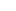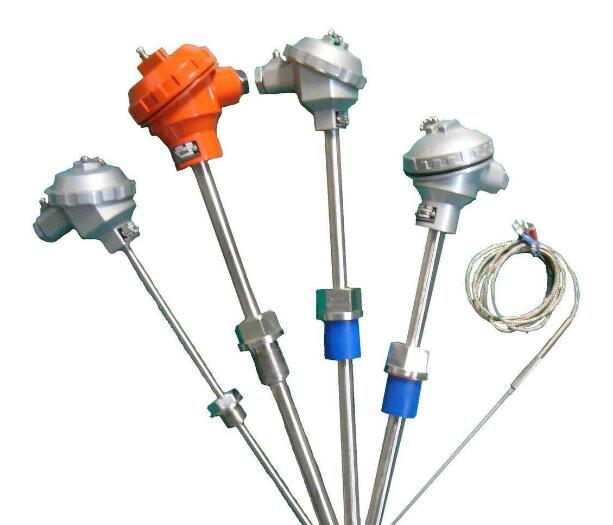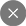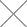Thermocouple manufacturer and supplier-Shanghai MKYDaires@cepai.com

##### SHANGHAI MKYD INSTRUMENT

Mobile:+86-17717522891
Wechat:17717522891
Tel:+86-21-54891500
Email：aires@cepai.com

### How does thermocouple work and thermocouple working principle2020-05-03

How does thermocouple work and thermocouple working principle

When there are two different conductors and semiconductors A and B TO form A loop, its both ends are connected, as long as the temperature of the two nodes is different, the end temperature of T, referred TO as the working end or hot end, the other end of the temperature of the TO, known as the free end (also known as the reference side) or the cold end, the back there is current in its path, which exist in the loop of electromotive force is called thermoelectric emfs.This phenomenon of electromotive force due to temperature difference is called seebeck effect.

There are two effects related to seebeck: first, when an electric current flows through the junction of two different conductors, heat is absorbed or given off (depending on the direction of the current), which is called the perlpost effect;Second, when an electric current flows through a conductor with a temperature gradient, the conductor absorbs or gives off heat (depending on the direction of the current relative to the temperature gradient), known as the Thomson effect.A combination of two different conductors or semiconductors is called a thermocouple.The thermoelectric potential EAB(T, T0) of the thermocouple is synthesized from the contact potential and the thermoelectric potential.Contact potential refers to the potential generated at the contact of two different conductors or semiconductors. This potential is related to the properties of the two conductors or semiconductors and the temperature at the contact point.Thermoelectric potential refers to the electric potential generated by the same conductor or semiconductor at both ends of different temperatures, which is only related to the properties of the conductor or semiconductor and the temperature at both ends, and has nothing to do with the length of the conductor, the size of the section, and the temperature distribution along its length direction.Both the contact potential and the thermoelectric potential are potential due to the different number of electrons concentrated at the end of the contact, and the thermoelectric potential measured by the thermocouple is a combination of the two.

When the circuit is disconnected, there is an electric potential delta V between a and b, the polarity and size of which are consistent with the thermoelectric potential in the circuit.It is also stipulated that at the cold end, when the current flows from A to B, A is called positive pole and B is called negative pole.Experiments show that when delta V is small, delta V is proportional to delta T.Define the differential thermoelectric potential of delta V to delta T as the thermoelectric potential rate, also known as seebeck coefficient.The sign and size of seebeck's coefficient depend on the thermoelectric characteristics of the two conductors that make up the thermocouple and the temperature difference of the junction.## INQUIRY US微信扫一扫 添加我为好友了解更多